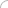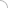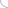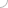# Stock and shares - aptitude test

Home > Aptitude test > Stock and shares

### 1) Find the number of shares that can be bought for Rs.8200 if the market value is Rs.20 each with brokerage being 2.5%.

a) 450
b) 500
c) 400
d) 410

Solution:

Cost of each share = (20 + 2.5% of 20) = Rs.20.5
Therefore, number of shares = 8200/20.5 = 400

### 2) Find the market value of the stock if 6% yields 10%.

a) 60
b) 70
c) 80
d) 100

Solution:

Let the investment be Rs.100 for an income of Rs.10
Therefore, for an income of Rs.6, the investment = 600/10 = Rs.60

### 3) What is the investment made if one invests in 15% stock at 50 and earns Rs.2000?

a) 8000
b) 7000
c) 5000
d) 6000

Solution:

To earn Rs.15, investment = Rs.50.
Hence, to earn Rs.1500, investment = (1500*50)/15
= Rs.5000

### 4) A company pays 12.5% dividend to its investors. If an investor buys Rs.50 shares and gets 25% on investment, at what price did the investor buy the shares?

a) 6.25
b) 25
c) 50
d) 12.5

Solution:

Dividend on 1 share = (12.5 * 50)/100 = Rs.6.25
Rs.25 is income on an investment of Rs.100
Rs.6.25 is income on an investment of Rs. (6.25 * 100)/25 = Rs.25

### 5) Which of the following is a better investment?

a) 10% stock at 80
b) 15% stock at 70
c) 12% stock at 75
d) All of the above

Solution:

For an investment of Rs.80, income = Rs.10
For an investment of Rs.100, income = Rs. (100 * 10)/80 = Rs.12.5
For an investment of Rs.70, income = Rs.15
For an investment of Rs.100, income = Rs. (100 * 15)/70 = Rs.21.43
For an investment of Rs.75, income = Rs.12
For an investment of Rs.100, income = Rs. (100 * 12)/75 = Rs.16Aptitude questions